# 1 Purpose

There are numerous programs out there for performing acoustic analysis, including several open-source options and R packages such as seewave and warbleR. Soundgen offers tools for visualizing audio (oscillograms, spectrograms, modulation spectra, self-similarity matrices), pitch tracking and formant analysis (both as standard functions and as interactive web apps), audio segmentation, as well as more specialized functions for estimating subjective loudness, roughness, acoustic novelty, surprisal, etc.

Many of the large variety of existing tools for acoustic analysis were designed with a particular type of sound in mind, usually human speech or bird songs. Soundgen was originally developed to work with human nonverbal vocalizations such as screams and laughs. These sounds are much harsher and noisier than ordinary speech, but they closely resemble vocalizations of other mammals. Acoustic analysis with soundgen may therefore be particularly useful for extracting a large number of acoustic predictors from collection of vocalizations of humans and other mammals, for example:

• describing the vocal repertoire of a species (clustering),
• using machine learning for acoustic classification,
• comparing different classes of sounds in terms of specific acoustic predictors.

The most relevant soundgen functions for acoustic analysis are:

• `analyze`: extracts a number of acoustic predictors such as pitch, harmonics-to-noise ratio, mean frequency, peak frequency, formants, roughness, intensity and loudness, etc. The output includes a summary per file, with each variable presented as mean / median / SD / …, as well as detailed statistics per STFT frame
• `pitch_app`: opens a shiny app in a web browser for manually correcting and exporting the pitch contours extracted by `analyze`
• `formant_app`: another web app, for annotating and manually correcting formant measurements provided by phonTools::findformants()
• `segment`: segments a long recording into individual syllables using spectral signal-noise separation
• `ssm`: produces a self-similarity matrix and calculates a novelty contour
• `getLoudness`: estimates the subjective loudness per STFT frame, in sone
• `modulationSpectrum`: calculates a joint temporal-spectral modulation spectrum and a measure of acoustic roughness
• `getRMS`: measures the root mean square amplitude of audio files
• `spectrogram`, `osc`: basic plots

TIP For a tour-de-force overview of alternatives together with highly accessible theoretical explanations of sound characteristics, see Sueur (2018) “Sound analysis and synthesis with R”

This vignette is designed to show how soundgen can be used effectively to perform acoustic analysis. It assumes that the reader is already familiar with key concepts of phonetics and bioacoustics.

# 2 Visualization

To demonstrate acoustic analysis in practice, let’s begin by generating a sound with a known pitch contour. To make pitch tracking less trivial and demonstrate some of its challenges, let’s add some noise, subharmonics, and jitter:

``library(soundgen)``
``## Loading required package: shinyBS``
``## Soundgen 2.4.0. Tips & demos on project's homepage: http://cogsci.se/soundgen.html``
``````s1 = soundgen(sylLen = 900, temperature = 0.001,
pitch = list(time = c(0, .3, .8, 1),
value = c(300, 900, 400, 1300)),
noise = c(-40, -20),
subFreq = 100, subDep = 20, jitterDep = 0.5,
plot = TRUE, ylim = c(0, 4))``````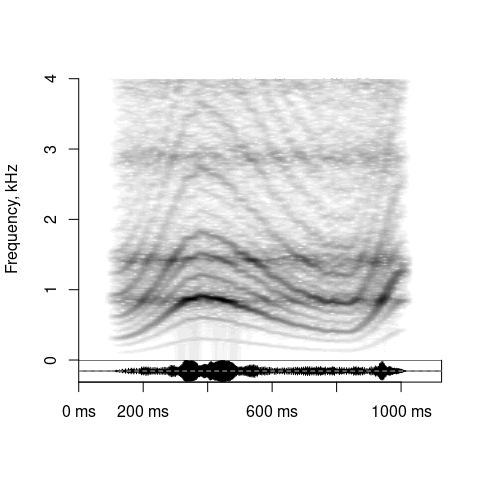``# playme(s1)  # to replay w/o re-synthesizing the sound``

Mathematically, digital audio is a time series - a long vector of real numbers representing sound pressure levels over time. This time series can be visualized in different domains:

1. Time domain. We just plot the original time series as an oscillogram. Function: `osc()`
``osc(s1, samplingRate = 16000, dB = TRUE)``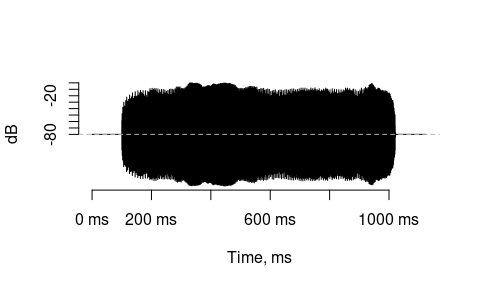``# Or just plain base R: plot(s1, type = 'l')``
1. Frequency domain. We can use a Fast Fourier Transform (FFT) to obtain the spectrum showing which frequencies are present in the signal. Functions: `seewave::spec()`, `seewave::meanspec()`. If we want to see how this frequency composition varies over time, we produce a spectrogram such as the plot above. The most common method for doing this is Short-Time Fourier Transform (STFT, function `spectrogram()`), but it is also possible to use a bank of bandpass filters (function `audSpectrogram()`) or the wavelet transform (e.g., function `wavelets::dwt()`).

Long-time average spectrum:

``seewave::meanspec(s1, f = 16000, dB = 'max0', main = 'Spectrum')``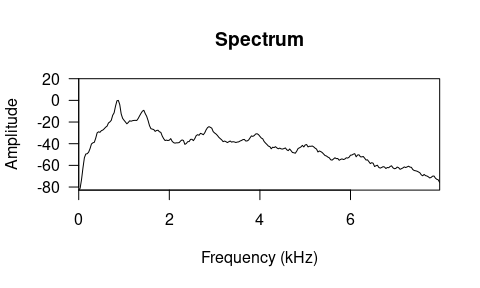Spectrogram with an oscillogram shown underneath:

``````spectrogram(
s1, samplingRate = 16000,
osc = 'dB',  # plot oscillogram in dB
heights = c(2, 1),  # spectro/osc height ratio
noiseReduction = .5,  # subtract the spectrum of noisy parts
brightness = -1,  # reduce brightness
contrast = .5,  # increase contrast
colorTheme = 'heat.colors',  # pick color theme
cex.lab = .75, cex.axis = .75,  # text size and other base graphics pars
grid = 5,  # lines per kHz; to customize, add manually with graphics::grid()
ylim = c(0, 5),  # always in kHzmain = 'Spectrogram')
main = 'Spectrogram'
)``````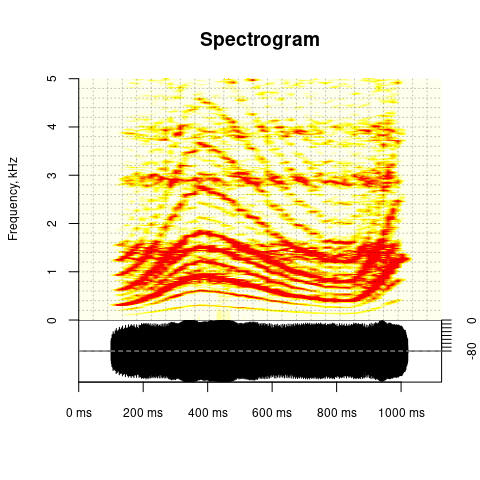``# see ?spectrogram for explanation of settings and more examples``

Auditory spectrogram of the same sound, obtained with a bank of filters, on the bark frequency scale :

``````audSpectrogram(s1, samplingRate = 16000,
nFilters = 128, step = 5,
colorTheme = 'terrain.colors',
main = 'Auditory spectrogram')``````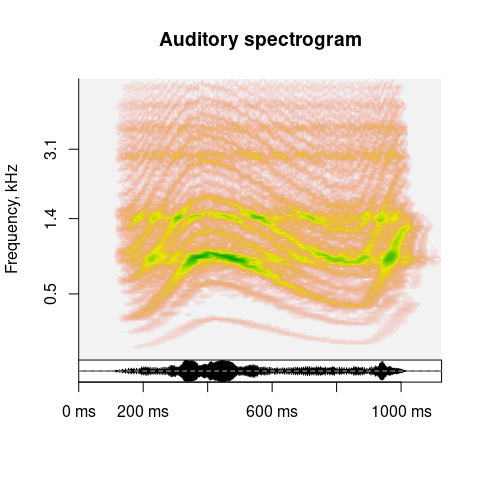1. Cepstral domain: taking a Fourier-flavored transformation one step further, we can also analyze what frequencies are present in the spectrum (used for pitch tracking in `analyze()`) or spectrogram (function `modulationSpectrum()` - more detail in section 7).
``````modulationSpectrum(s1, samplingRate = 16000, colorTheme = 'seewave',
plot = TRUE, main = 'Modulation spectrum')``````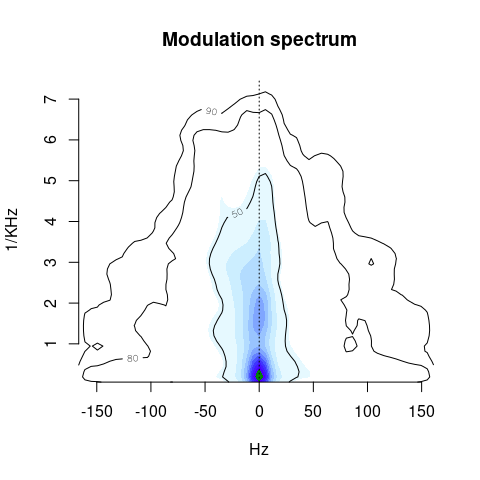1. Other. Less common visual representations of audio include neurologically inspired spectro-temporal receptive fields, gammatones, etc. For example, soundgen can plot self-similarity matrices that represent spectral changes over time (more detail in section 8):
``ssm(s1, samplingRate = 16000, main = 'Self-similarity matrix')``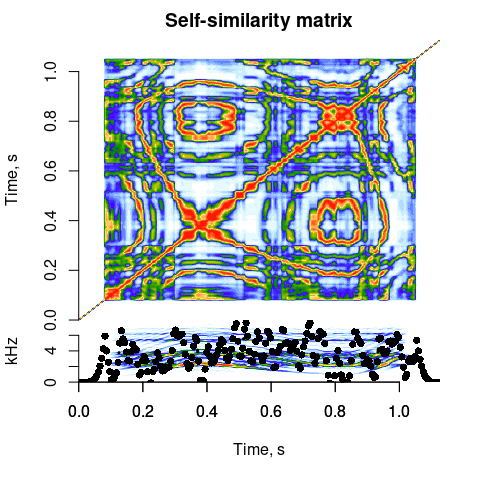TIP: most soundgen functions for acoustic analysis and visualization accept as input both a single sound and a path to folder, in which case all the audio files in this folder are processed in one go. For example, copy a few recordings into ‘~/Downloads/temp’ and run `spectrogram('~/Downloads/temp', savePlots = '~/Downloads/temp/plots')` –> a separate .png file will be saved for each recording. Likewise, `analyze(‘path_to_folder’) analyzes all audio files in the target folder.

# 3 Acoustic analysis with `analyze`

Soundgen has specialized functions for particular types of acoustic analysis. However, for convenience, most of them are called internally by `analyze()`, which provides basic spectral descriptives, pitch tracking, formant analysis, as well as estimates of roughness, subjective loudness, novelty, etc.

## 3.1 Basic principles

At the heart of acoustic analysis with soundgen is the short-time Fourier transform (STFT): we look at one short segment of sound at a time (one STFT frame), analyze its spectrum using Fast Fourier Transform (FFT), and then move on to the next - perhaps overlapping - frame. To analyze a sound with default settings and plot its spectrogram, all we need to specify is its sampling rate (the default in soundgen is 16000 Hz):

``````a1 = analyze(s1, samplingRate = 16000, plot = TRUE, ylim = c(0, 4))
# a1\$detailed  # many acoustic predictors measured for each STFT frame
median(a1\$detailed\$pitch, na.rm = TRUE)  # our estimate of median pitch``````
``##  713.077``
``# Pitch postprocessing is stochastic (see below), so the contour may vary.``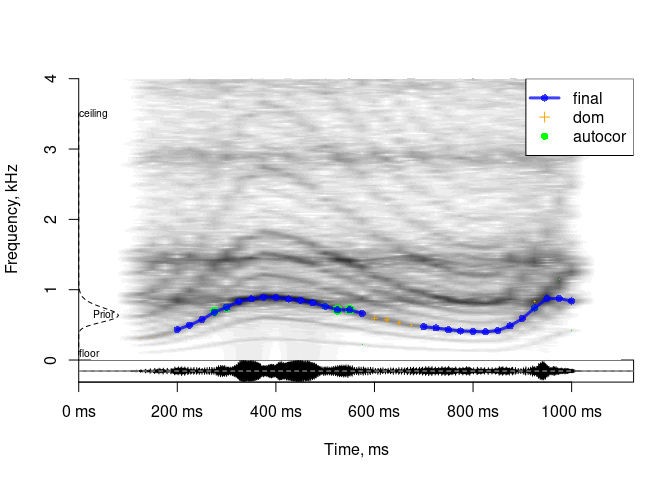There are several key parameters that control the behavior of STFT and affect all extracted acoustic variables. The same parameters serve as arguments to `spectrogram`. As a result, you can immediately see what frame-by-frame input you have fed into the algorithm for acoustic analysis by visually inspecting the produced spectrogram. If you can hear f0, but can’t see individual harmonics in the spectrogram, the pitch tracker probably will not see them, either, and will therefore fail to detect f0 correctly. The first remedy is thus to adjust STFT settings, using the spectrogram for visual feedback:

• `windowLength`: the length of sliding STFT window. Longer windows (e.g., 40 - 50 ms) improve frequency resolution at the expense of time resolution, so they are good for detecting relatively low, slowly changing f0. Shorter windows (e.g., 5 - 10 ms) improve time resolution at the expense of frequency resolution, so they are good for visualizing formants or for tracking high-frequency, rapidly changing f0 as in bird chirps or dolphin whistles.
• `step`: the step of sliding STFT window. For example, if `windowLength = 50` and `step = 25`, each time we move the analysis frame, there is a 50% overlap with the previous frame. Short steps improve time resolution while maintaining relatively high frequency resolution at the price of processing time. For noisy recordings, pitch contour may actually be more accurate with relatively large steps and more smoothing.
• `wn`: the type of windowing function used to taper the analysis frame during STFT. In practice the windowing function doesn’t seem to have a major effect on the result, as long as you choose something reasonable like gaussian, hanning, or bartlett.
• `zp`: zero-padding. You can use a short STFT window and improve its frequency resolution by padding each frame with zeroes. This is a computational trick for improving frequency resolution while maintaining relatively high time resolution.
• `silence`: frames with root mean square (RMS) amplitude below silence threshold are not analyzed at all. Quiet frames are harder to analyze, because their signal-to-noise ratio is lower. As a result, we want to strike a good balance. Setting `silence` too low (close to 0) produces a lot of garbage, as the algorithm tries to analyze frames that are essentially just background noise without any signal. Setting `silence` too high (close to 1) excludes too many perfectly good frames, misrepresenting the signal. In soundgen `silence` is dynamically updated: it can never be lower than specified, but it may be raised to the minimum root mean square amplitude of all frames, if this minimum is higher than `silence`. This ensures that empty frames are not analyzed in recordings with unusually high levels of steady background noise (e.g., microphone hiss).

## 3.2 Spectral descriptives

Apart from pitch tracking, `analyze` calculates and returns several acoustic characteristics from each non-silent STFT frame:

• `time`: time of the middle of each frame (ms)
• `duration`: total duration (s)
• `duration_noSilence`: duration from the beginning of the first non-silent STFT frame to the end of the last non-silent STFT frame, s (NB: depends strongly on `windowLength` and `silence` settings)
• `amDep`: depth of amplitude modulation, dB (estimated by `modulationSpectrum`, the arguments to which are passed in `roughness = list()`)
• `amFreq`: frequency of amplitude modulation, Hz (estimated by `modulationSpectrum`, the arguments to which are passed in `roughness = list()`)
• `ampl`: root mean square of amplitude per frame, calculated as `sqrt(mean(frame ^ 2))`
• `dom`: lowest dominant frequency band (Hz) (see “Pitch tracking methods / Dominant frequency”)
• `entropy`: Weiner entropy of the spectrum of the current frame. Close to 0: pure tone or tonal sound with nearly all energy in harmonics; close to 1: white noise
• `f1_freq`, `f1_width`, …: the frequency and bandwidth of the first `nFormants` formants per STFT frame, as calculated by `phonTools::findformants` with default settings
• `flux`: feature-based flux, the rate of change in acoustic features such as pitch, HNR, etc.; unitless (0 = none)
• `harmEnergy`: the amount of energy in upper harmonics, namely the ratio of total spectral energy above 1.25 x f0 to the total spectral energy below 1.25 x f0 (dB)
• `harmHeight`: how high harmonics reach in the spectrum, based on the best guess at pitch (or the manually provided pitch values): see `soundgen:::harmHeight` for details
• `HNR`: harmonics-to-noise ratio (dB), a measure of harmonicity returned by `soundgen:::getPitchAutocor()` (see “Pitch tracking methods / Autocorrelation”). If HNR = 0 dB, there is as much energy in harmonics as in noise
• `loudness`: subjective loudness in sone, assuming a certain sound pressure level (takes into account the energy in different frequency bands as well as the sensitivity of human ears to different frequencies); see `getLoudness()` and the section on Loudness below for details
• `novelty`: spectral novelty - a measure of how variable the spectrum is on a particular time scale, as estimated by `ssm()`
• `peakFreq`: the frequency with maximum spectral energy below `cutFreq` (Hz)
• `roughness`: the amount of spectrotemporal modulation in the “roughness” zone of frequencies (estimated by `modulationSpectrum`, the arguments to which are passed in `roughness = list()`)
• `quartile25`, `quartile50`, `quartile75`: the 25th, 50th, and 75th quantiles of the spectrum below `cutFreq` (Hz) for VOICED frames
• `specCentroid`: the center of gravity of the frame’s spectrum below `cutFreq`, first spectral moment (Hz)
• `specSlope`: the slope of linear regression fit to the spectrum below `cutFreq`
• `voiced`: is the current STFT frame voiced? TRUE / FALSE

TIP: many of these descriptors are calculated separately for the entire sound and only for the voiced frames, resulting in extra output variables like “amplVoiced”.

## 3.3 Custom spectral descriptives

The function `soundgen::analyze` returns a few spectral descriptives that make sense for nonverbal vocalizations, but additional predictors may be useful for other applications (bird songs, non-biological sounds, etc.). Many popular spectral descriptors are mathematically trivial to derive - all you need is the spectrum for each STFT frame, or perhaps even the average spectrum of the entire sound. Here is how you can get these spectra.

For the average spectrum of an entire sound, go no further than `seewave::spec` or `seewave::meanspec`:

``````spec = seewave::spec(s1, f = 16000, plot = FALSE)  # FFT of the entire sound
avSpec = seewave::meanspec(s1, f = 16000, plot = FALSE)  # STFT followed by averaging
# either way, you get a dataframe with two columns: frequencies and their strength
``````##            x            y
## [1,] 0.00000 7.223228e-05
## [2,] 0.03125 8.804839e-05
## [3,] 0.06250 2.258169e-04
## [4,] 0.09375 7.685260e-04
## [5,] 0.12500 2.247701e-03
## [6,] 0.15625 3.340433e-03``````

If you are interested in how the spectrum changes over time, extract frame-by-frame spectra - for example, with `spectrogram(..., output = 'original')`:

``````spgm = spectrogram(s1, samplingRate = 16000, output = 'original', plot = FALSE)
# rownames give you frequencies in KHz, colnames are time stamps in ms
str(spgm)``````
``````##  num [1:400, 1:78] 4.53e-05 3.51e-05 1.75e-05 1.07e-05 7.17e-06 ...
##  - attr(*, "dimnames")=List of 2
##   ..\$ : chr [1:400] "0" "0.02" "0.04" "0.06" ...
##   ..\$ : chr [1:78] "0" "15" "30" "45" ...``````

Let’s say you are working with frame-by-frame spectra and want to calculate skewness, the 66.6th percentile, and the ratio of energy above/below 500 Hz. Before you go hunting for a piece of software that returns exactly those descriptors, consider this. Once you have normalized the spectrum to add up to 1, it basically becomes a probability density function (pdf), so you can summarize it in the same way as you would any other distribution of a random variable. Look up the formulas you need and just do the raw math:

``````# Transform spectrum to pdf (all columns should sum to 1):
spgm_norm = apply(spgm, 2, function(x) x / sum(x))
# Set up a dataframe to store the output
out = data.frame(skew = rep(NA, ncol(spgm)),
quantile66 = NA,
ratio500 = NA)
# Process each STFT frame
for (i in 1:ncol(spgm_norm)) {
# Absolute spectrum for this frame
df = data.frame(
freq = as.numeric(rownames(spgm_norm)),  # frequency (kHz)
d = spgm_norm[, i]                       # density
)
# plot(df, type = 'l')

# Skewness (see https://en.wikipedia.org/wiki/Central_moment)
m = sum(df\$freq * df\$d)  # spectral centroid, kHz
out\$skew[i] = sum((df\$freq - m)^3 * df\$d)

# 66.6th percentile (2/3 of density below this frequency)
out\$quantile66[i] = df\$freq[min(which(cumsum(df\$d) >= 2/3))]  # in kHz

# Energy above/below 500 Hz
out\$ratio500[i] = sum(df\$d[df\$freq >= .5]) / sum(df\$d[df\$freq < .5])
}``````
``````## Warning in min(which(cumsum(df\$d) >= 2/3)): no non-missing arguments to min;
## returning Inf``````
``summary(out)``
``````##       skew            quantile66       ratio500
##  Min.   : 0.01562   Min.   :0.020   Min.   :  0.0015
##  1st Qu.: 2.70894   1st Qu.:1.160   1st Qu.: 19.3570
##  Median : 2.93577   Median :1.400   Median : 36.8679
##  Mean   : 3.31513   Mean   :1.204   Mean   : 79.8904
##  3rd Qu.: 3.09830   3rd Qu.:1.460   3rd Qu.:114.0162
##  Max.   :13.70326   Max.   :2.420   Max.   :347.6595
##  NA's   :1          NA's   :1       NA's   :1``````

If you need to do this analysis repeatedly, just wrap the code into your own function that takes a wav file as input and returns all these spectral descriptives. You can also save the actual spectra of different sound files and add them up to obtain an average spectrum across multiple sound files, work with cochleograms instead of raw spectra (check out `tuneR::melfcc`), etc. Be your own boss!

## 3.4 Pitch tracking

Fundamental frequency (f0) or its perceptual equivalent - pitch - is both highly salient to listeners and notoriously difficult to measure accurately. The approach followed by soundgen’s pitch tracker is to use several different estimates of f0, each of which is better suited to certain types of sounds. You can use any pitch tracker individually, but their output is also automatically integrated and postprocessed so as to generate the best overall estimate of frame-by-frame pitch. Autocorrelation is needed to calculate the harmonics-to-noise ratio (HNR) of an STFT frame, and then this information is used to adjust the expectations of the cepstral and spectral algorithms. In particular, if autocorrelation suggests that the pitch is high, confidence in cepstral estimates is attenuated; and if autocorrelation suggests that HNR is low, thresholds for spectral peak detection are raised, making spectral pitch estimates more conservative.

The plot below shows a spectrogram of the sound with overlaid pitch candidates generated by five different methods (listed in `pitchMethods`), with a very vague prior - that is, with no specific expectations regarding the true range of pitch values. The size of each point shows the certainty of estimation: smaller points are calculated with lower certainty and have less weight when all candidates are integrated into the final pitch contour (blue line).

``````a = analyze(s1, samplingRate = 16000, priorSD = 24,
pitchMethods = c('autocor', 'cep', 'dom', 'spec', 'hps', 'zc'),
plot = TRUE, ylim = c(0, 4))``````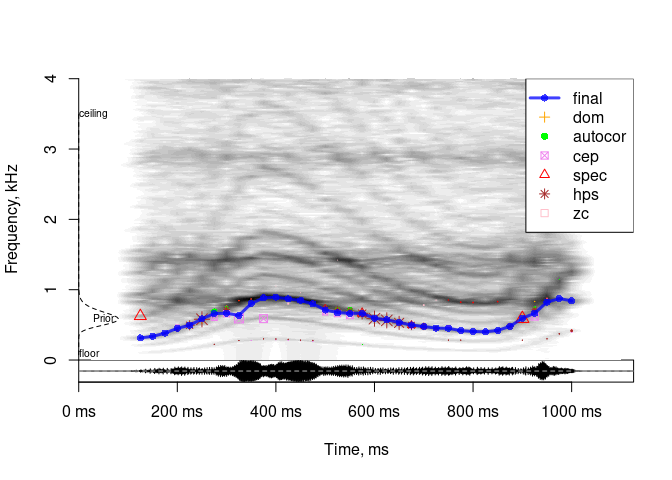Different pitch tracking methods have their own pros and cons. Cepstrum is helpful for speech but pretty useless for high-frequency whistles or screams, harmonic product spectrum (hps) is easily misled by subharmonics (as in this example), lowest dominant frequency band (dom) can’t handle low-frequency wind noise or missing fundamental, etc. The default is to use “dom” and “autocor” as the most generally applicable, but you can experiment with all methods and check which ones perform best with the specific type of audio that you are analyzing. Each method can also be fine-tuned (see below), but first it is worth considering the general pitch-related settings.

### 3.4.1 General settings

`analyze` has a few arguments that affect all methods of pitch tracking:

• `entropyThres`: all non-silent frames are analyzed to produce basic spectral descriptives. However, pitch tracking is both computationally costly and can be misleading if applied to obviously voiceless frames. To define what an “obviously voiceless” frame is, we set some cutoff value of Weiner entropy, above which we don’t want to even try pitch tracking. To disable this feature and track pitch in all non-silent frames, set `entropyThres = NULL`.
• `pitchFloor`, `pitchCeiling`: absolute thresholds for pitch candidates. No values outside these bounds will be considered.
• `priorMean` and `priorSD` specify the mean and sd of prior distribution describing our prior knowledge about the most likely pitch values. If `priorAdapt = TRUE`, this user-defined prior is only applies to the first pass of optimizing pitch contours, after which the first-pass contour becomes the new prior for the second pass. Both of these priors work by scaling the certainties associated with particular pitch candidates. If you are working with a single type of sound, such as speech by a male speaker or cricket sounds, specifying a strong prior can greatly improve the quality of the resulting pitch contour. When batch-processing a large number of sounds, the recommended approach is to set a vague, but still mildly informative prior.

Note that `priorMean` is specified in Hz and `priorSD` in semitones. For example, if we expect f0 values of about 300 Hz plus-minus half an octave (6 semitones), a prior can be defined as `priorMean = 300, priorSD = 6`. For convenience, the prior can be plotted with `getPrior`:

``````par(mfrow = c(1, 2))
# default prior in soundgen
getPrior(priorMean = 300, priorSD = 6)
# narrow peak at 2 kHz
getPrior(priorMean = 2000, priorSD = 1)
par(mfrow = c(1, 1))``````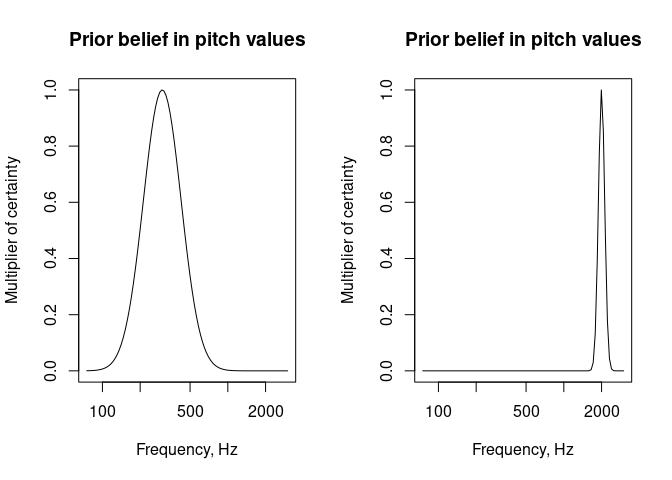TIP The final pitch contour can still pass through low-certainty candidates, so the prior is a soft alternative (or addition) to the inflexible bounds of `pitchFloor` and `pitchCeiling` But the prior has a major impact on pitch tracking, so it is by default shown in every plot

• `nCands`: maximum number of pitch candidates to use per method. This doesn’t affects `dom` pitch candidates (only a single value of the lowest dominant frequency is used regardless).
• `minVoicedCands`: minimum number of pitch candidates that have to be defined to consider a frame voiced. It defaults to ‘autom’, which means 2 if `dom` is among the candidates and 1 otherwise. The reason is that `dom` is usually defined, even if the frame is clearly voiceless, so we want another pitch candidate in addition to `dom` before we classify the frame as voiced.

### 3.4.2 Pitch tracking methods

Having looked at the general settings, it is time to consider the theoretical principles behind each pitch tracking method, together with arguments to `analyze` that can be used to tweak each one.

#### 3.4.2.1 Autocorrelation

Time domain: pitch by autocorrelation, PRAAT, `pitchAutocor`.

This is an R implementation of the algorithm used in the popular open-source program PRAAT (Boersma, 1993). The basic idea is that a harmonic signal correlates with itself most strongly at a delay equal to the period of its fundamental frequency (f0). Peaks in the autocorrelation function are thus treated as potential pitch candidates. The main trick is to choose an appropriate windowing function and adjust for its own autocorrelation. Compared to other methods implemented in soundgen, pitch estimates based on autocorrelation appear to be particularly accurate for relatively high values of f0. The settings that control `pitchAutocor` are:

• `autocorThres`: voicing threshold, defaults to 0.7. This means that peaks in the autocorrelation function have to be at least 0.7 in height (1 = perfect autocorrelation). A lower threshold produces more false positives (f0 is detected in voiceless, noisy frames), whereas a higher threshold produces more accurate values f0 at the expense of failing to detect f0 in noisier frames.
• `autocorSmooth`: the width of smoothing interval (in bins) for finding peaks in the autocorrelation function. If left NULL, it defaults to 7 for sampling rate 44100 and smaller odd numbers for lower sampling rate.
• `autocorUpsample`: upsamples the autocorrelation function in high frequencies in order to improve the resolution of analysis.
• `autocorBestPeak`: amplitude of the lowest best candidate relative to the absolute maximum of the autocorrelation function.

To use only autocorrelation pitch tracking, but with lower-than-default voicing threshold and more candidates, we can do something like this (prior is disabled so as not to influence the certainties of different pitch candidates):

``````a = analyze(s1, samplingRate = 16000,
plot = TRUE, ylim = c(0, 2), priorMean = NA, osc = FALSE,
pitchMethods = 'autocor',
pitchAutocor = list(autocorThres = .45,
# + plot pars if needed
col = 'green'),
nCands = 3)``````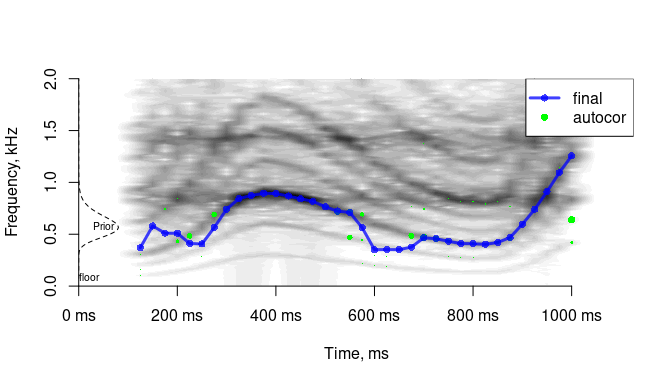#### 3.4.2.2 Dominant frequency

Frequency domain: the lowest dominant frequency band, `dom`.

If the sound is harmonic and relatively noise-free, the spectrum of a frame typically has little energy below f0. It is therefore likely that the first spectral peak is in fact f0, and all we have to do is choose a reasonable threshold to define what counts as a peak. Naturally, there are cases of missing f0 and misleading low-frequency noises. Nevertheless, this simple estimate is often surprisingly accurate, and it may be our best shot when the vocal cords are vibrating in a chaotic fashion (deterministic chaos). For example, sounds such as roars lack clear harmonics but are perceived as voiced, and the lowest dominant frequency band (which may also be the first or second formant) often corresponds to perceived pitch.

The settings that control `dom` are:

• `domThres` (defaults to 0.1, range 0 to 1): to find the lowest dominant frequency band, we look for the lowest frequency with amplitude at least `domThres`. This key setting has to be high enough to exclude accidental low-frequency noises, but low enough not to miss f0. As a result, the optimal level depends a lot on the type of sound analyzed and recording conditions.
• `domSmooth` (defaults to 220 Hz): the width of smoothing interval (Hz) for finding the lowest spectral peak. The idea is that we are less likely to hit upon some accidental spectral noise and find the lowest harmonic (or the lowest spectral band with significant power) if we apply some smoothing to the spectrum of an STFT frame, in this case a moving median.

For the sound we are trying to analyze, we can increase `domSmooth` and/or raise `domThres` to ignore the subharmonics and trace the true pitch contour:

``````a = analyze(s1,  samplingRate = 16000,
plot = TRUE, ylim = c(0, 2), priorMean = NA, osc = FALSE,
pitchMethods = 'dom',
pitchDom = list(domThres = .1, domSmooth = 500, cex = 1.5))``````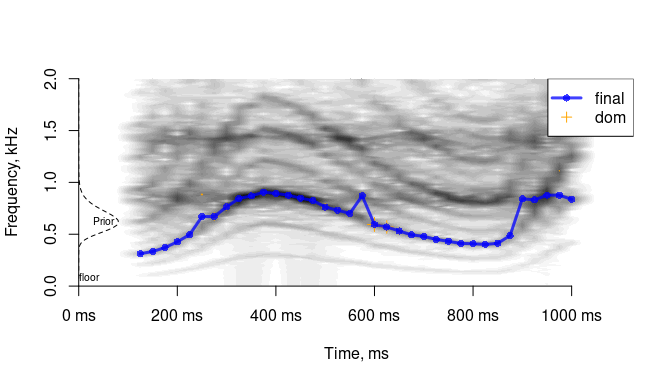#### 3.4.2.3 Cepstrum

Frequency domain: pitch by cepstrum, `pitchCep`.

Cepstrum is the FFT of log-spectrum. It may be a bit challenging to wrap one’s head around, but the main idea is quite simple: just as FFT is a way to find periodicity in a signal, cepstrum is a way to find periodicity in the spectrum. In other words, if the spectrum contains regularly spaced harmonics, its FFT will contain a peak corresponding to this regularity. And since the distance between harmonics equals the fundamental frequency, this cepstral peak gives us f0. Actually, in soundgen the FFT is applied to raw spectrum, not log-spectrum, since it appears to produce better results. Cepstrum is not very useful when f0 is so high that the spectrum contains only a few harmonics, so soundgen automatically discounts the contribution of high-frequency cepstral estimates.

The settings that control `pitchCep` are:

• `cepThres`: voicing threshold (defaults to 0.3).
• `cepSmooth`: the width of smoothing interval (in Hz) for finding peaks in the cepstrum. If left NULL, it defaults to 31 bins for sampling rate 44100 and smaller odd numbers for lower values of sampling rate.
• `cepZp` (defaults to 0): zero-padding of the spectrum used for cepstral pitch detection (points). Zero-padding may improve the precision of cepstral pitch detection, but it also slows down the algorithm.
``````a = analyze(s1, samplingRate = 16000,
plot = TRUE, ylim = c(0, 2), priorMean = NA, osc = FALSE,
pitchMethods = 'cep',
pitchCep = list(cepThres = .3),
nCands = 2)``````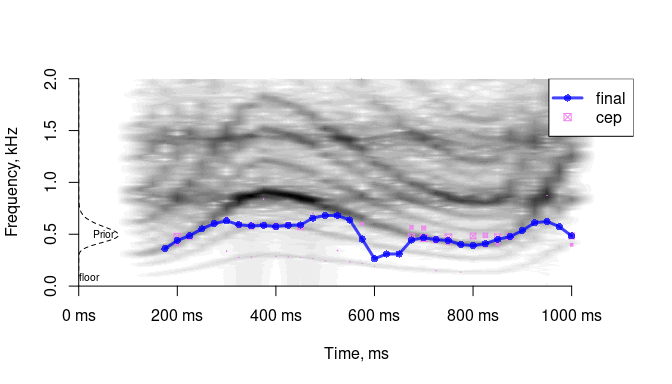#### 3.4.2.4 Spectral harmonics

Frequency domain: common factor of harmonics or ratios of harmonics, `pitchSpec`.

All harmonics are multiples of the fundamental frequency. If we can detect several harmonics, we can therefore find f0 as the highest common ratio of harmonics (method = “commonFactor”). Alternatively, we can exploit the fact that the ratio of two neighboring harmonics is predictably related to their rank relative to f0 (method = “BaNa”). For example, `(3 * f0) / (2 * f0) = 1.5`, so if we find two harmonics in the spectrum that have a ratio of exactly 1.5, it is likely that f0 is half the lower one (Ba et al., 2012).

The settings that control `pitchSpec` are:

• `specMethod`: “commonFactor” = highest common factor of putative harmonics, “BaNa” = ratio of putative harmonics
• `specRatios`: for method = “commonFactor”, the number of harmonics AND integer fractions to consider
• `specMerge` (0 to Inf semitones, defaults to 1): pitch candidates within `specMerge` semitones are merged with boosted certainty. Since the idea behind the spectral pitch tracker is that multiple harmonic ratios should converge on the same f0, we have to decide what counts as “the same” f0
• `specThres` (0 to 1, defaults to 0.1): voicing threshold for pitch candidates suggested by the spectral method. The scale is 0 to 1, as usual, but it is the result of a rather arbitrary normalization. The “strength” of spectral pitch candidates is basically calculated as a sigmoid function of the number of common factors or harmonic ratios that imply (nearly) the same f0 value. Setting `specThres` too low may produce garbage, while setting it too high makes the spectral method excessively conservative.
• `specPeak` (0 to 1, defaults to 0.25), `specHNRslope` (0 to Inf, defaults to 0.8): when looking for putative harmonics in the spectrum, the threshold for peak detection is calculated as `specPeak * (1 - HNR * specHNRslope)`. For noisy sounds the threshold is high to avoid false sumharmonics, while for tonal sounds it is low to catch weak harmonics. If `HNR` (harmonics-to-noise ratio) is not known, say if we have disabled the autocorrelation pitch tracker or if it returns NA for a frame, then the threshold defaults to simply `specPeak`. This key parameter strongly affects how many pitch candidates the spectral method suggests.
• `specSmooth` (0 to Inf, defaults to 150 Hz): the width of window for detecting peaks in the spectrum, in Hz. You may want to adjust it if you are working with sounds with a specific f0 range, especially if it is unusually high or low compared to human sounds.
• `specSinglePeakCert`: (0 to 1, defaults to 0.4) if a`pitchSpec` candidate is calculated based on a single harmonic ratio (as opposed to several ratios converging on the same candidate), its weight (certainty) is taken to be `specSinglePeakCert`. This mainly has implications for how much we trust spectral vs. other pitch estimates.
``````a = analyze(s1, samplingRate = 16000,
plot = TRUE, ylim = c(0, 2), priorMean = NA, osc = FALSE,
pitchMethods = 'spec',
pitchSpec = list(specPeak = .025, specSmooth = 400, cex = 2))``````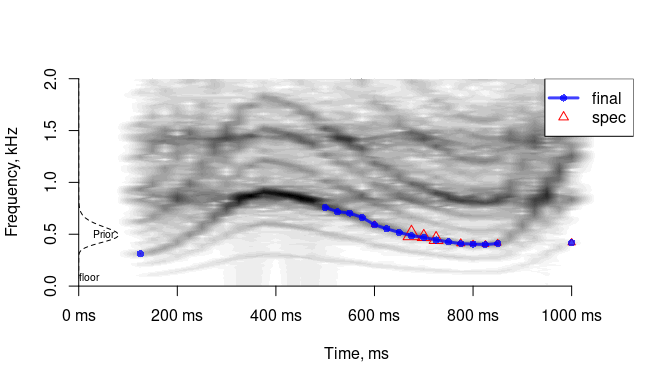#### 3.4.2.5 Harmonic product spectrum

Frequency domain: `pitchHps`.

This is a simple spectral method based on downsampling the spectrum several times and multiplying the resulting spectra. This emphasizes the lowest harmonic present in the signal, which is hopefully f0. By definition, this method is easily misled by subharmonics (additional harmonics between the main harmonics of f0), but it can be useful in situations when the subharmonic frequency is actually of interest.

The settings that control `pitchHps` are:

• `hpsThres` (0 to 1, defaults to 0.3): voicing threshold for pitch candidates suggested by `hps` method
• `hpsNum` (defaults to 5): the number of times the spectrum is downsampled. Increasing the number improves sensitivity in the sense that the method converges on the lowest harmonic, which is generally (but not always) desirable
• `hpsNorm`: the amount of inflation of hps pitch certainty (0 = none). Because the downsampled spectra are multiplied, the height of the resulting peak tends to be rather low; `hpsNorm` (defaults to 2, 0 = none) compensates for it, otherwise this method would have very low confidence compared to other pitch trackers
• `hpsPenalty` (defaults to 2, 0 = none): the amount of penalizing hps candidates in low frequencies (0 = none). HPS doesn’t perform very well at low frequencies, so the certainty in low-frequency candidates is attenuated
``````a = analyze(s1, samplingRate = 16000,
plot = TRUE, ylim = c(0, 2), priorMean = NA, osc = FALSE,
pitchMethods = 'hps',
pitchHps = list(hpsNum = 2, # try 8 or so to measure subharmonics
hpsThres = .2))``````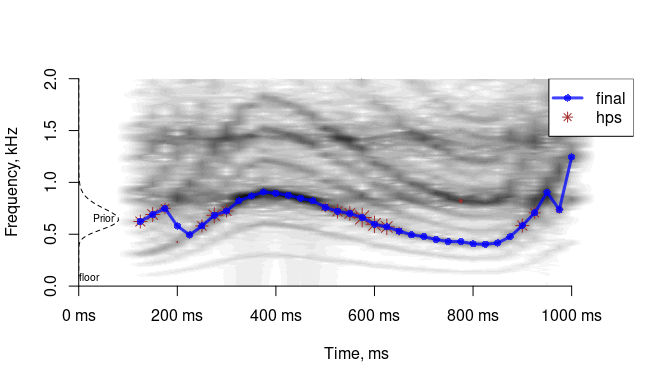#### 3.4.2.6 Zero crossings

Time domain: `pitchZc`.

This is a very simple and fast time-domain method based on detecting zero crossings after bandpass-filtering the audio. This method only works if f0 is strong enough (e.g., well below the first formant in speech) and can be suitable for rapidly analyzing long speech recordings. Note that a relatively low `pitchCeiling` must be specified, essentially just above the upper values of f0.

The settings that control `pitchZc` are:

• `zcThres` (0 to 1, defaults to 0.1): pitch candidates with certainty below this value are treated as noise and set to NA
• `zcWin` (odd integer > 3): certainty in pitch candidates depends on how stable pitch is over zcWin glottal cycles
``````a = analyze(s1, samplingRate = 16000,
plot = TRUE, ylim = c(0, 2), priorMean = NA, osc = FALSE,
pitchMethods = 'zc',
pitchCeiling = 600,  # higher pitch values blend with F1
pitchZc = list(zcWin = 3, zcThres = 0))``````